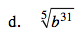### Home > CALC > Chapter 1 > Lesson 1.2.2 > Problem1-41

1-41.
1. Wei Kit knows that roots can be re-written using exponents. Study his examples below:

2. EXAMPLES: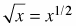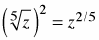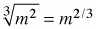3. Use Wei Kit's method to rewrite the following radicals using exponents. Homework Help ✎

1.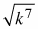2.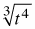3.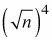4.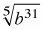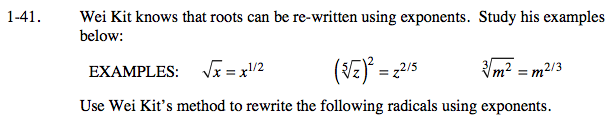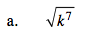$\small k^{\frac{1}{7}}$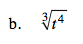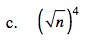$\text{Recall: } \left(\sqrt{n}\right)^4=\left(n^{\frac{1}{2}}\right)^{4}$